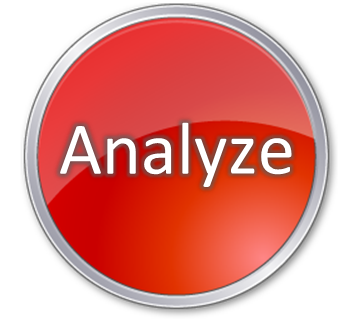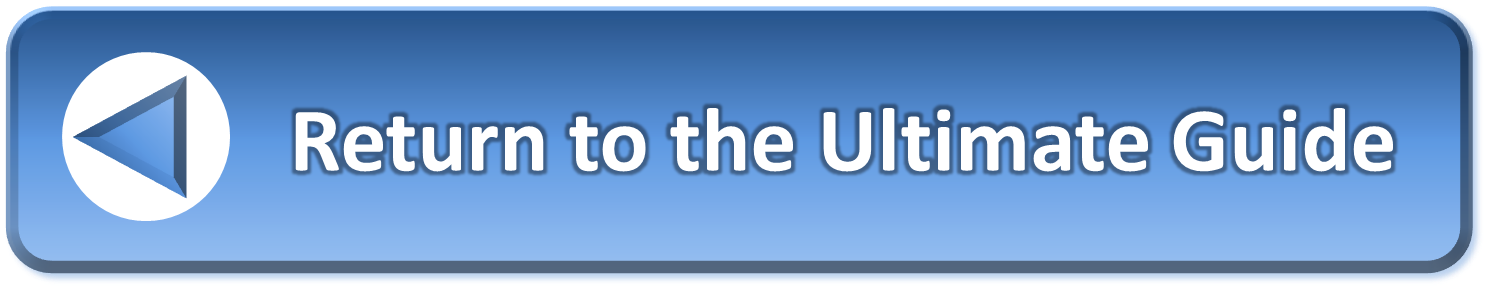Not a member yet?What

Preliminary list of standard 'What' questions.

• What is the real problem?
• What are the key questions that need to be answered?
• What do you know about the problem?
• What don’t you know about the problem?
• What are the essential elements of the problem?
• What is at the “heart” of the problem?
• What is most critical area of concern?
• What part of this situation is most important to focus on?
• What part stands out and demands attention?
• What are the parts of the problem?
• What are the sub-problems?
• What is the problem a part of?
• What belongs to it?
• What elements of the problem are related to one another?
• What are in the areas adjacent to the situation?
• What can be changed about it?
• What cannot be changed?
• What are their strengths?
• What do you do well?
• What advantages do you have?
• What are their weaknesses?
• What could be improved?
• What are others doing better?
• What do others see as your weaknesses?
• What are the interesting trends?
• What opportunities are available to you?
• What is the biggest opportunity?
• What are the risk factors?
• What are the threats?
• What are the obstacles?
• What is preventing you from getting what you want?
• What obstacles do you face?
• What may possibly go wrong?
• What are some of the difficulties that could occur?
• What weakness could lead to catastrophic failure?
• What is the worst imaginable thing that could occur?
• What are the constraints?
• What restrictions must you accept to solve the problem?
• What is your competition doing?
• What changes in technology threaten your position?
• What changes in cash flow would threaten your position?
• What values underlie it?
• What is the value base of the problem?
• What sensory perceptions can be observed (i.e. see, hear, touch, smell, and taste)?
• What do other people see as the problem?
• What will it be like if the problem is solved?
• What will it be like if it isn’t solved?
• What are the good points of a bad situation?
• What are the bad points of a good situation?
• What do you like about the problem?
• What do you dislike about it?
• What do you feel is most important about the problem?
• What do you feel is least important about it?
• What would increase your motivation and enthusiasm?
• What makes you angry, tense, or frustrated about the problem?
• What makes you really excited about solving the problem?
• What outcomes would be inspiring?
• What do you want?
• What do you need?
• What are the rules or regulations?
• What are the financial constraints?
• What are the other resource limitations?
• What are the paradoxes in the situation?
• What else do you need to know?
• What data are important?
• What data might be grouped together?
• What patterns do you see in the data?
• What does the system do to convert inputs into outputs?
• What assumptions are you making about the problem?
• What are the consequences of having this problem?
• What happened before it?
• What happened after it?
• What happens at the same time?
• What is the context of it?
• What is the context o that context?
• What is the irritant we see? Is it the actual problem or merely a symptom?
• What can be substituted?
• What can be eliminated?
• What can be combined with it?
• What can be altered?
• What if it were exaggerated?
• What can be rearranged?
• What can be reversed?